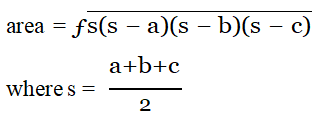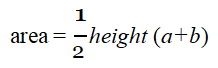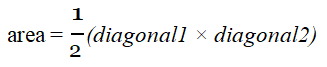#### Example Programs

Program 

Design a class to overload a function compare() as follows:

(a) void compare(int,int) - to compare tow integer values and print the greater of the tow integers.

(b) void compare(char,char) - to compare the numeric value of tow character with higher numeric value.

(c) void compare(String,String) - to compare the length of the tow strings and print the longer of the two.

Program 

Design a class to overload a function polygon() as follows:

(i) void polygon(int n, char ch) - with one integer argument and one character type argument that draws a filled square of side n using the character stored in ch.

(ii) void polygon(int x, int y) - with two integer arguments that draws a filled rectangle of length x and breadth y, using the symbol ‘@’

(iii) void polygon() - with no argument that draws a filled triangle shown below.

Sample I/O

(i) Input value of n = 2, ch=’O’

Output:

OO

OO

(ii) Input value of x = 2, y = 5

Output:

@@@@@

@@@@@

(iii) Output:

*

**

***

Program 

Design a class to overload a function area() as follows:

1. double area(double a, double b, double c) with three double arguments, returns the area of a scalene triangle using the formula:2. double area(int a, int b, int height) with three integer arguments, returns the area of a trapezium using the formula:3. double area(double diagonal1, double diagonal2) with two double arguments, returns the area of a rhombus using the formula: